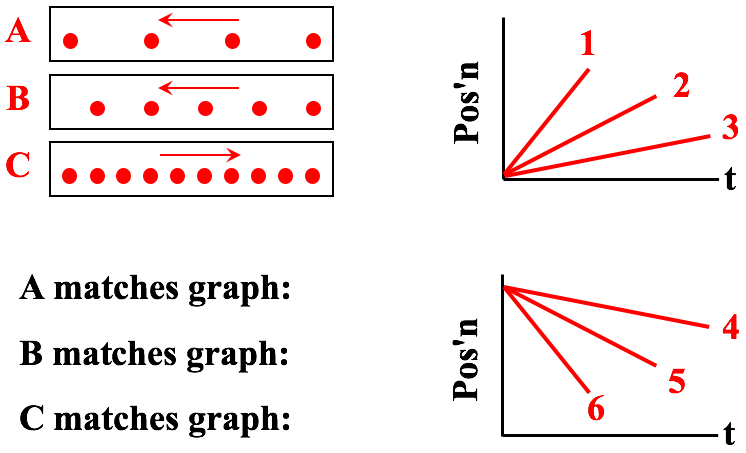## Position Time Graphs

The Position Time Graphs - Conceptual Analysis Concept Builder is comprised of 46 questions that are grouped into 14 different Question Groups and spread across three different activities. The three activities each have a slightly different emphasis. The first activity - Words and Graphs - focuses on the skill of associating the features of a position-time graph with the characteristics of an object's motion. The second activity - Speed Rankings - focuses on the skill of comparing speeds of objects based on the magnitude of the slope of their lines on a position-time graph. The third activity - Dots and Graphs - has students inspecting the dot diagram for three different motions and associating each motion with one of six lines on a position-time graph.  Questions are grouped with questions in the same group requiring a very similar skill or motion type. If a learner misses a question within Question Group 1, then another question within the same group will be presented to the learner at a later time.

The three activities in this Concept Builder are summarized as follows:

• Words and Graphs: Question Groups 1-6
• Speed Rankings: Question Groups 7-19
• Dots and Graphs: Question Groups 11-14

The questions and graphs used in this Concept Builder are shown below. Teachers are encouraged to view the questions in order to judge which activity is most appropriate for their classes. For instance, teachers of honors classes may find that all questions are appropriate. On the other hand, teachers of lower level or middle school classes may find that the Dots and Graphs activity is a bit too difficult for the bulk of their students.

The Physics Classroom grants teachers and other users the right to print these questions for private use. Users are also granted the right to copy the text and modify it for their own use. However, this document should not be uploaded to other servers for distribution to and/or display by others. The Physics Classroom website should remain the only website or server from which this document is distributed or displayed. We also provide a PDF that teachers can use under the same conditions. We have included a link to the PDF near the bottom of this page.

Position Time Graphs
Conceptual Analysis

Activity 1: Words and Graphs
Question Group 1
Question #1
Five stages - labeled A, B, C, D, and E - of an object's motion are represented by the position-time graph below. During which stage(s) is the object at rest?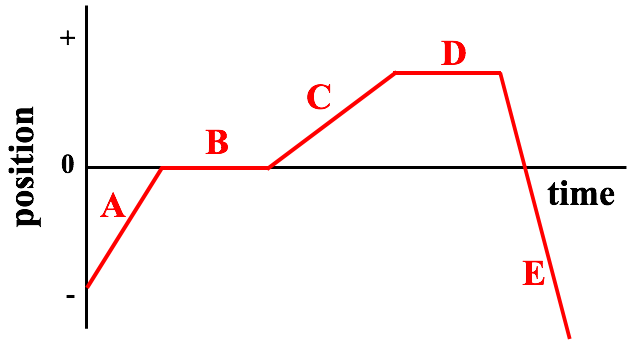Question #2
Five stages - labeled A, B, C, D, and E - of an object's motion are represented by the position-time graph below. During which stage(s) is the object at rest?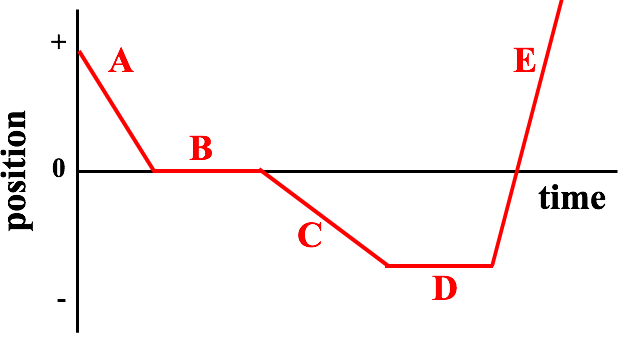Question #3
Five stages - labeled A, B, C, D, and E - of an object's motion are represented by the position-time graph below. During which stage(s) is the object at rest?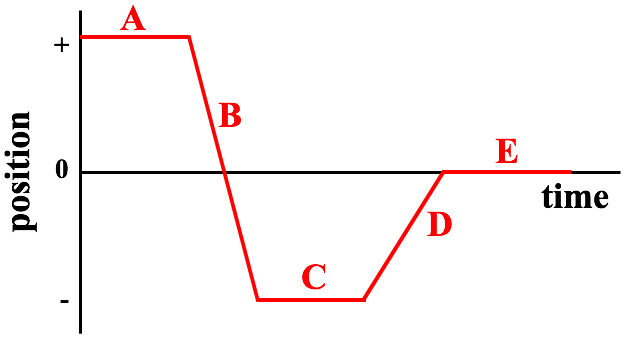Question Group 2
Question #4
Five stages - labeled A, B, C, D, and E - of an object's motion are represented by the position-time graph below. During which stage(s) is the object moving with a constant speed?Question #5
Five stages - labeled A, B, C, D, and E - of an object's motion are represented by the position-time graph below. During which stage(s) is the object moving with a constant speed?Question #6
Five stages - labeled A, B, C, D, and E - of an object's motion are represented by the position-time graph below. During which stage(s) is the object moving with a constant speed?Question Group 3
Question #7
Five stages - labeled A, B, C, D, and E - of an object's motion are represented by the position-time graph below. During which stage(s) does the object have a changing velocity?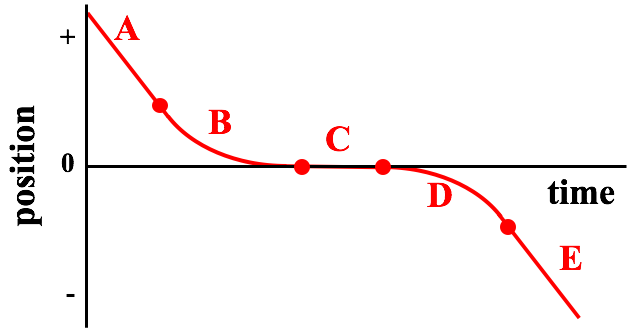Question #8
Five stages - labeled A, B, C, D, and E - of an object's motion are represented by the position-time graph below. During which stage(s) does the object have a changing velocity?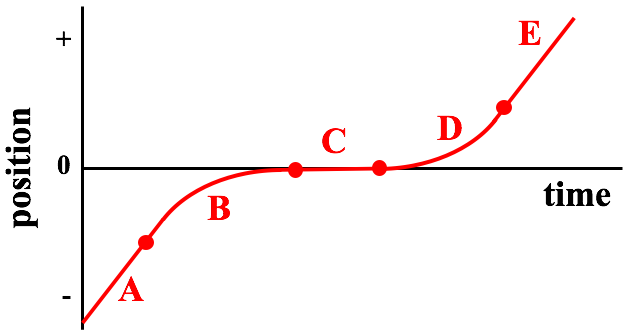Question #9
Five stages - labeled A, B, C, D, and E - of an object's motion are represented by the position-time graph below. During which stage(s) does the object have a changing velocity?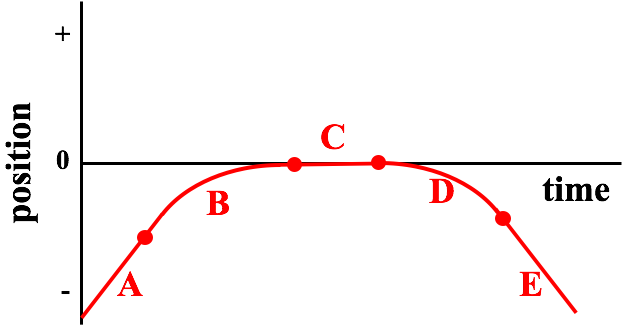Question Group 4
Question #10
Five stages - labeled A, B, C, D, and E - of an object's motion are represented by the position-time graph below. During which stage(s) does the object have a positive velocity?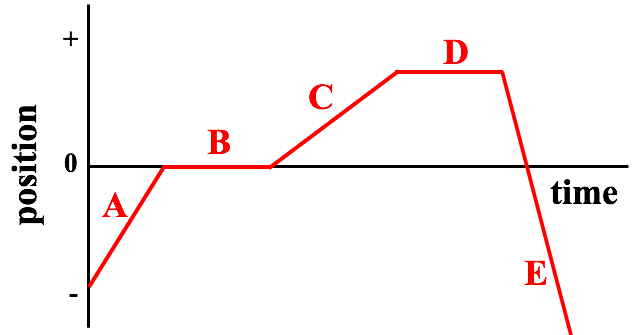Question #11
Five stages - labeled A, B, C, D, and E - of an object's motion are represented by the position-time graph below. During which stage(s) does the object have a positive velocity?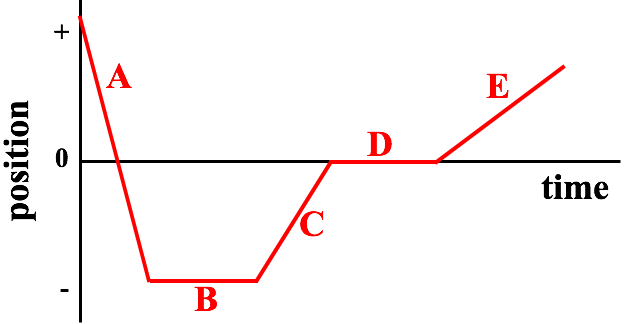Question #12
Five stages - labeled A, B, C, D, and E - of an object's motion are represented by the position-time graph below. During which stage(s) does the object have a negative velocity?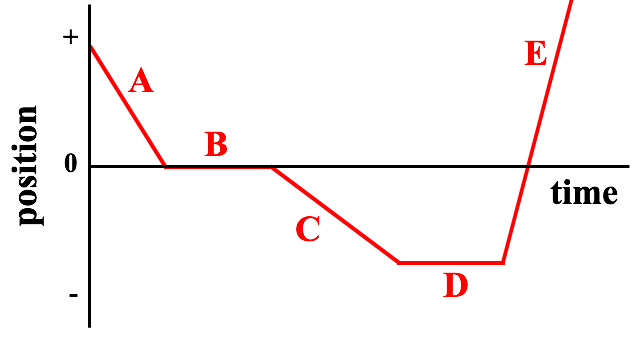Question #13
Five stages - labeled A, B, C, D, and E - of an object's motion are represented by the position-time graph below. During which stage(s) does the object have a negative velocity?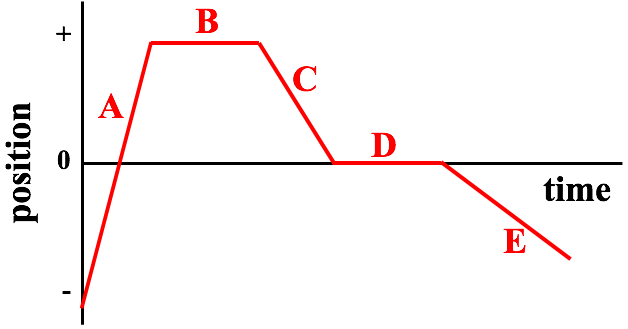Question Group 5
Question #14
Five stages - labeled A, B, C, D, and E - of an object's motion are represented by the position-time graph below. During which stage(s) is the object moving away from its starting point?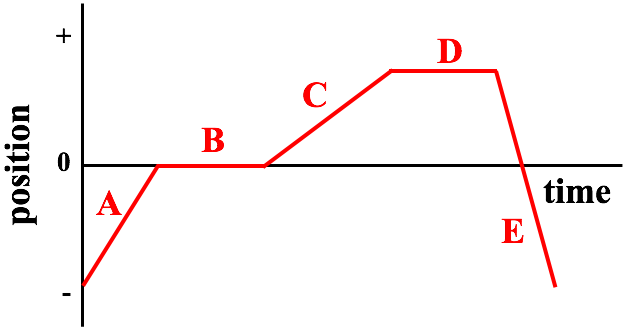Question #15
Five stages - labeled A, B, C, D, and E - of an object's motion are represented by the position-time graph below. During which stage(s) is the object moving away from its starting point?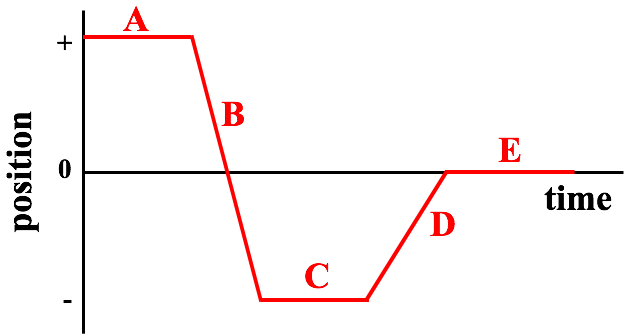Question #16
Five stages - labeled A, B, C, D, and E - of an object's motion are represented by the position-time graph below. During which stage(s) is the object moving towards its starting point?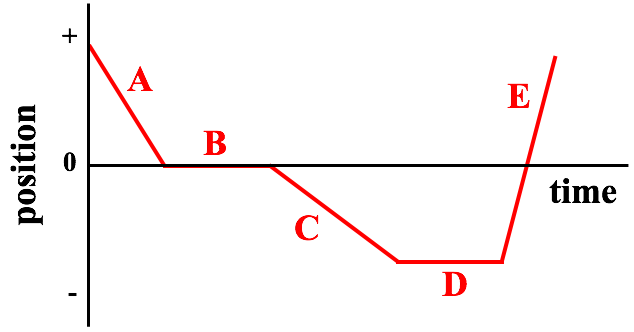Question #17
Five stages - labeled A, B, C, D, and E - of an object's motion are represented by the position-time graph below. During which stage(s) is the object moving towards its starting point?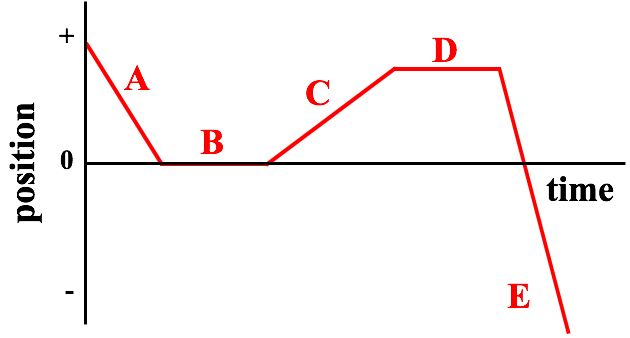Question Group 6
Question #18
Five stages - labeled A, B, C, D, and E - of an object's motion are represented by the position-time graph below. During which stage is the object moving with the greatest speed?Question #19
Five stages - labeled A, B, C, D, and E - of an object's motion are represented by the position-time graph below. During which stage is the object moving with the greatest speed?Question #20
Five stages - labeled A, B, C, D, and E - of an object's motion are represented by the position-time graph below. During which stage is the object moving with the smallest speed?Question #21
Five stages - labeled A, B, C, D, and E - of an object's motion are represented by the position-time graph below. During which stage is the object moving with the smallest speed?Question Group 7
Question #22
The motions of Objects A, B, and C are represented on a position-time graph. Observe their lines and rank the speeds of Objects A, B, and C from slowest to fastest.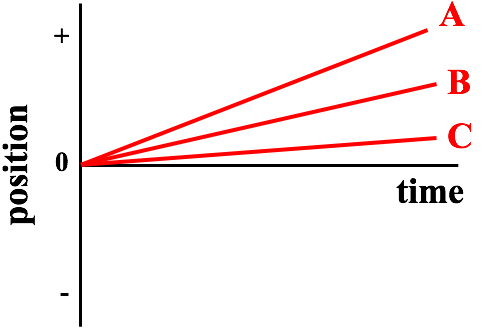Question #23
The motions of Objects A, B, and C are represented on a position-time graph. Observe their lines and rank the speeds of Objects A, B, and C from slowest to fastest.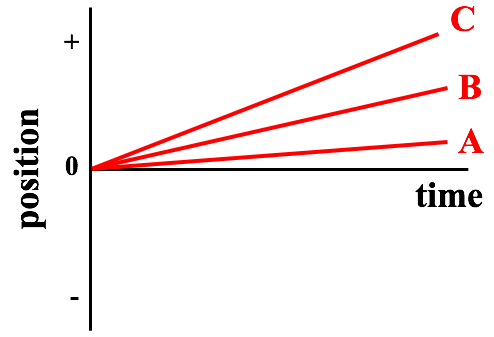Question #24
The motions of Objects A, B, and C are represented on a position-time graph. Observe their lines and rank the speeds of Objects A, B, and C from slowest to fastest.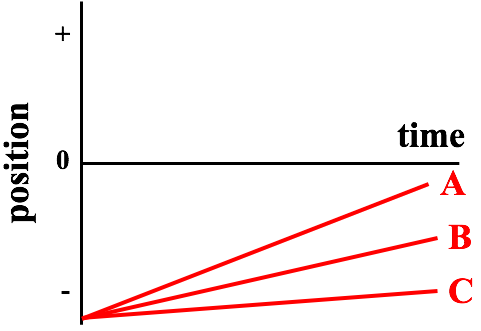Question #25
The motions of Objects A, B, and C are represented on a position-time graph. Observe their lines and rank the speeds of Objects A, B, and C from slowest to fastest.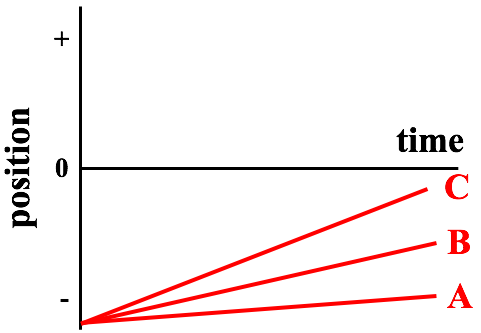Question Group 8
Question #26
The motions of Objects A, B, and C are represented on a position-time graph. Observe their lines and rank the speeds of Objects A, B, and C from slowest to fastest.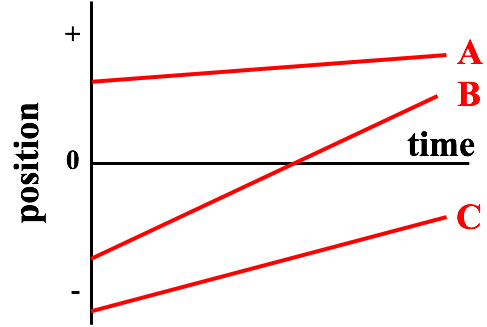Question #27
The motions of Objects A, B, and C are represented on a position-time graph. Observe their lines and rank the speeds of Objects A, B, and C from slowest to fastest.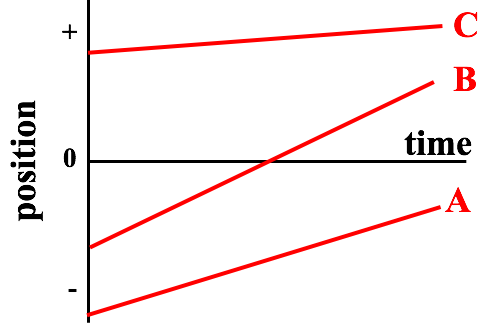Question #28
The motions of Objects A, B, and C are represented on a position-time graph. Observe their lines and rank the speeds of Objects A, B, and C from slowest to fastest.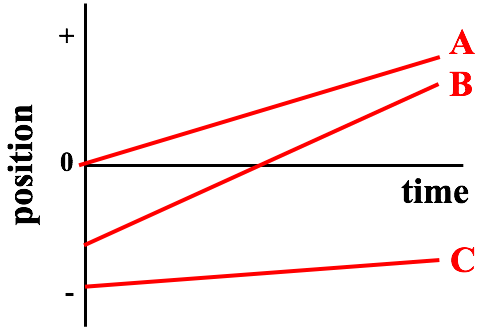Question Group 9
Question #29
The motions of Objects A, B, and C are represented on a position-time graph. Observe their lines and rank the speeds of Objects A, B, and C from slowest to fastest.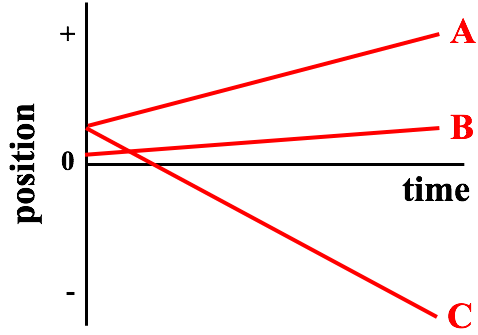Question #30
The motions of Objects A, B, and C are represented on a position-time graph. Observe their lines and rank the speeds of Objects A, B, and C from slowest to fastest.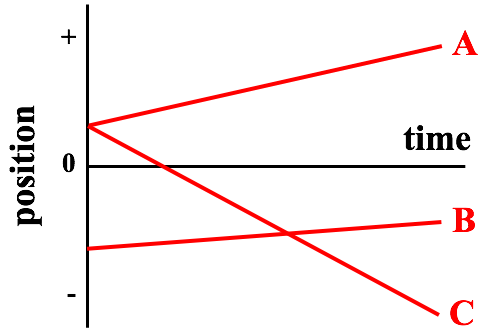Question #31
The motions of Objects A, B, and C are represented on a position-time graph. Observe their lines and rank the speeds of Objects A, B, and C from slowest to fastest.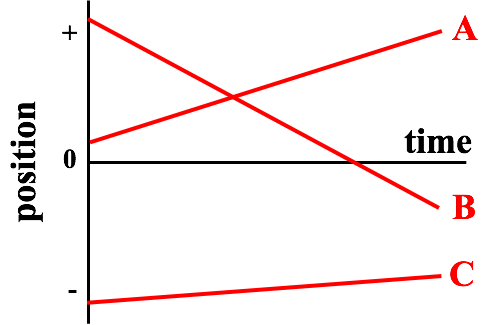Question Group 10
Question #32
The motions of Objects A, B, and C are represented on a position-time graph. Observe their lines and rank the speeds of Objects A, B, and C from slowest to fastest.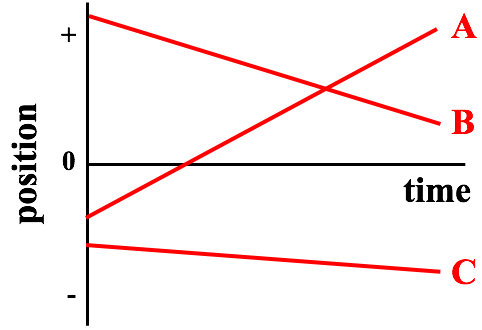Question #33
The motions of Objects A, B, and C are represented on a position-time graph. Observe their lines and rank the speeds of Objects A, B, and C from slowest to fastest.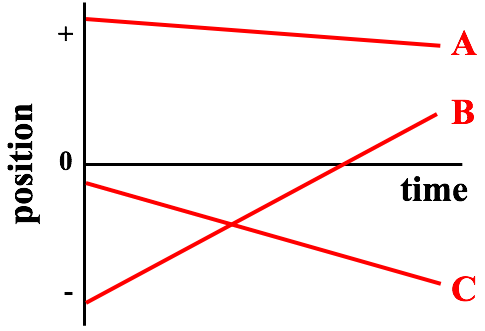Question #34
The motions of Objects A, B, and C are represented on a position-time graph. Observe the lines and rank the speeds of Objects A, B, and C from slowest to fastest.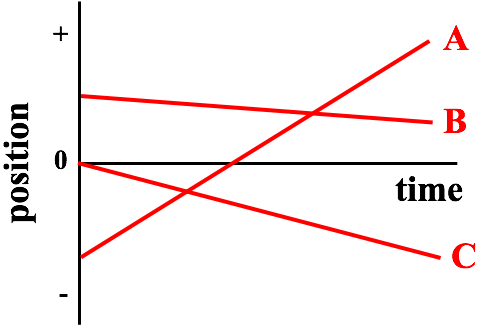Activity 3:  Dots and Graphs
Question Group 11
Question #35
Consider the dot diagrams below for Objects A, B, and C. The arrow represents the direction of travel. Match the motion of Objects A, B, and C to one of the lines on the graph.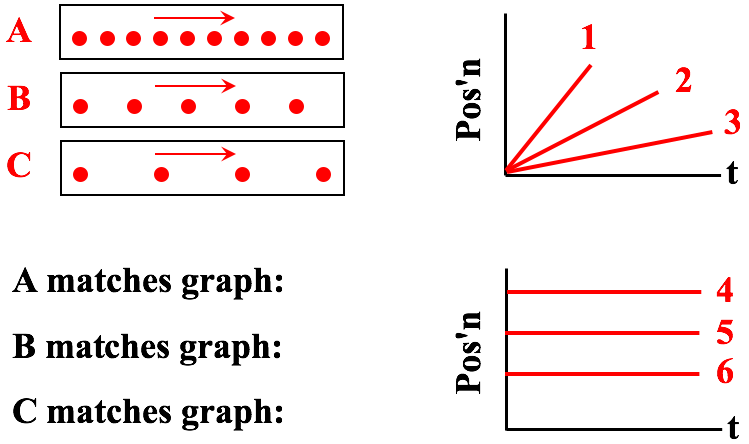Question #36
Consider the dot diagrams below for Objects A, B, and C. The arrow represents the direction of travel. Match the motion of Objects A, B, and C to one of the lines on the graph.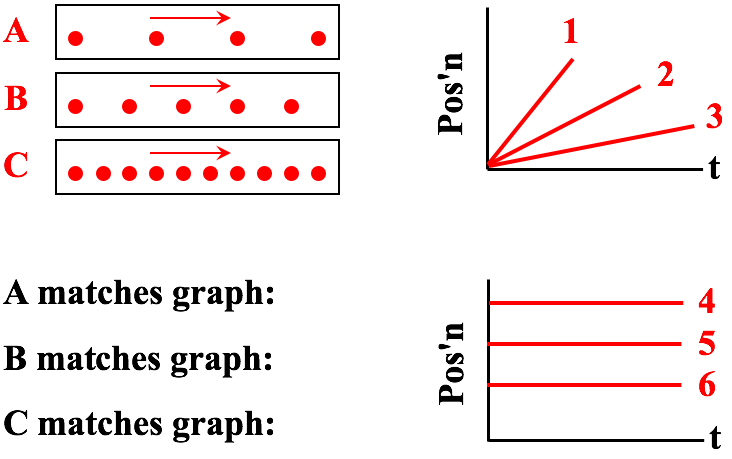Question #37
Consider the dot diagrams below for Objects A, B, and C. The arrow represents the direction of travel. Match the motion of Objects A, B, and C to one of the lines on the graph.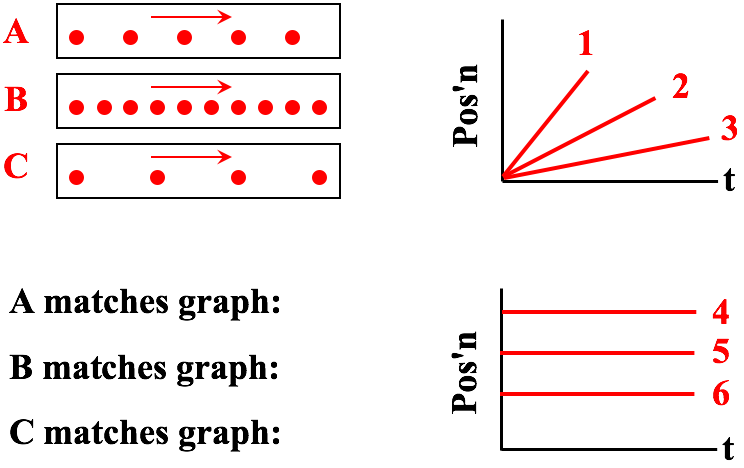Question Group 12
Question #38
Consider the dot diagrams below for Objects A, B, and C. The arrow represents the direction of travel. Match the motion of Objects A, B, and C to one of the lines on the graph.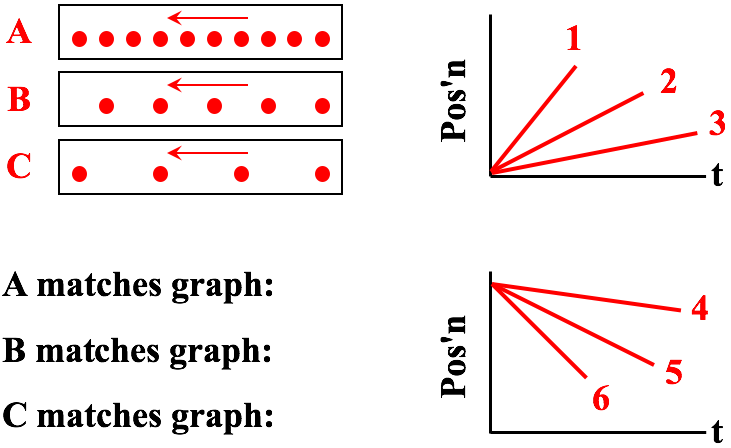Question #39
Consider the dot diagrams below for Objects A, B, and C. The arrow represents the direction of travel. Match the motion of Objects A, B, and C to one of the lines on the graph.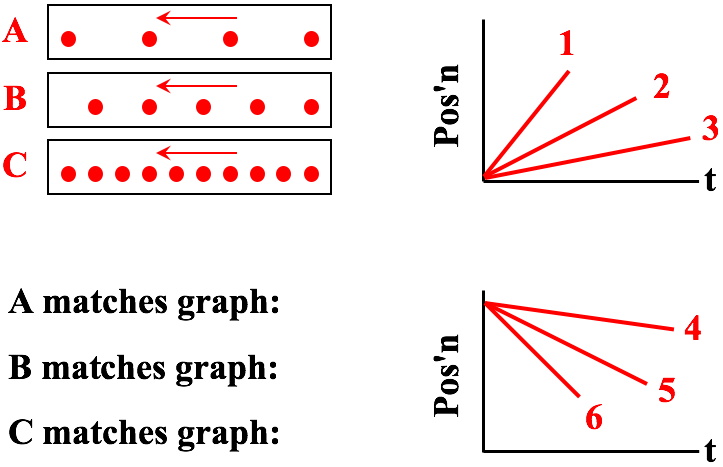Question #40
Consider the dot diagrams below for Objects A, B, and C. The arrow represents the direction of travel. Match the motion of Objects A, B, and C to one of the lines on the graph.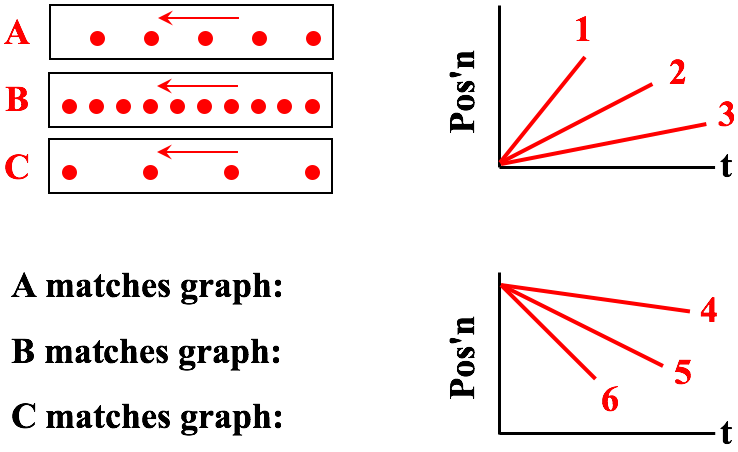Question Group 13
Question #41
Consider the dot diagrams below for Objects A, B, and C. The arrow represents the direction of travel. Match the motion of Objects A, B, and C to one of the lines on the graph.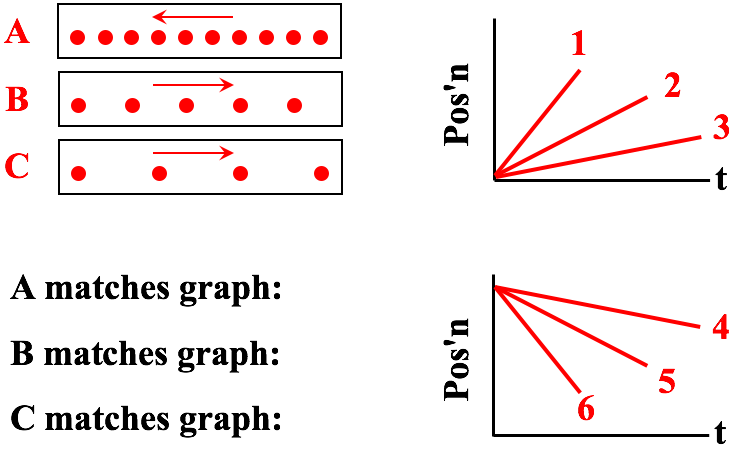Question #42
Consider the dot diagrams below for Objects A, B, and C. The arrow represents the direction of travel. Match the motion of Objects A, B, and C to one of the lines on the graph.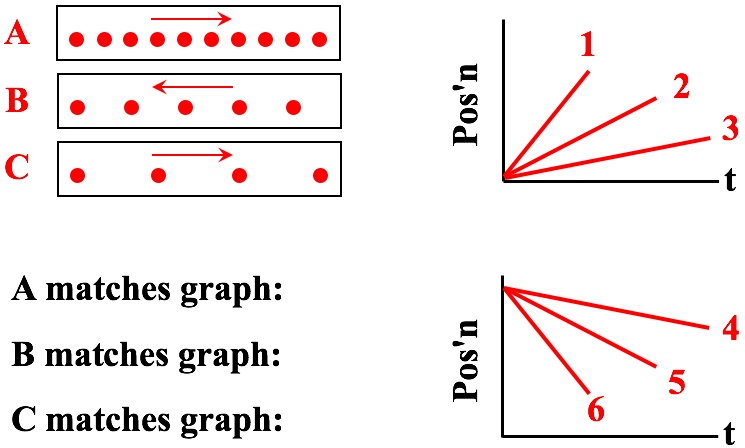Question #43
Consider the dot diagrams below for Objects A, B, and C. The arrow represents the direction of travel. Match the motion of Objects A, B, and C to one of the lines on the graph.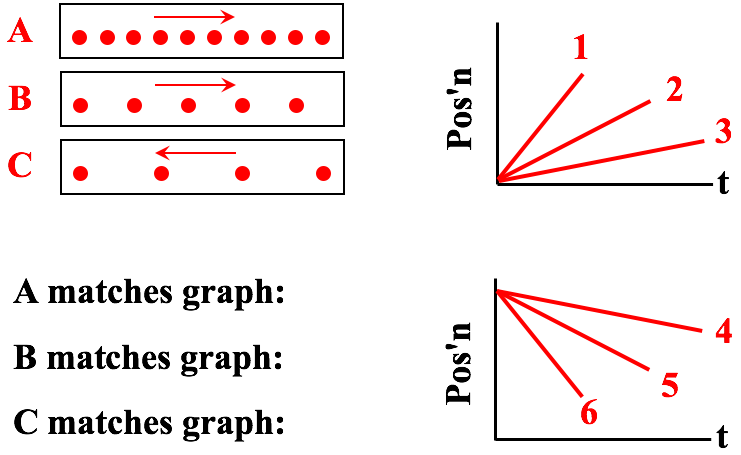Question Group 14
Question #44
Consider the dot diagrams below for Objects A, B, and C. The arrow represents the direction of travel. Match the motion of Objects A, B, and C to one of the lines on the graph.Question #45
Consider the dot diagrams below for Objects A, B, and C. The arrow represents the direction of travel. Match the motion of Objects A, B, and C to one of the lines on the graph.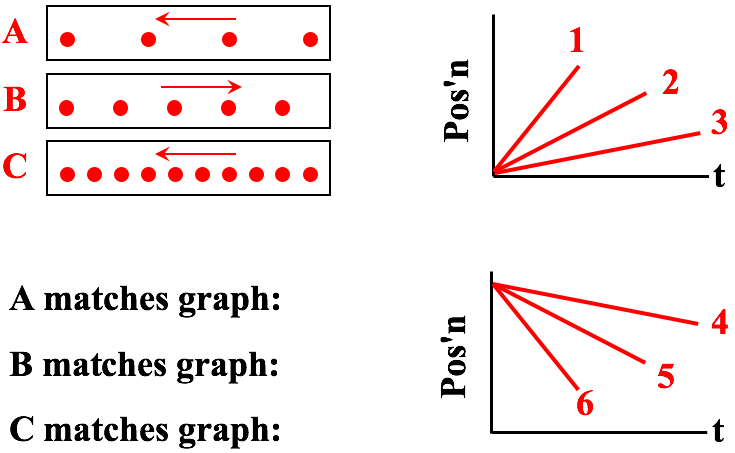Question #46
Consider the dot diagrams below for Objects A, B, and C. The arrow represents the direction of travel. Match the motion of Objects A, B, and C to one of the lines on the graph.